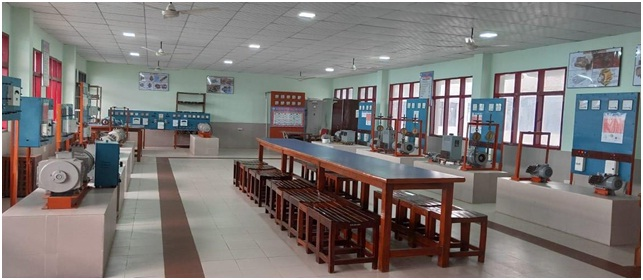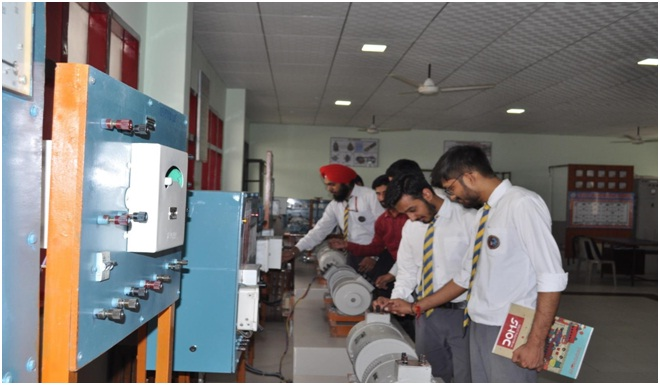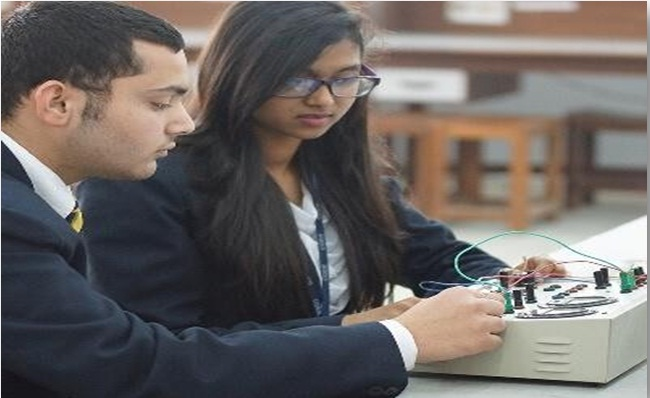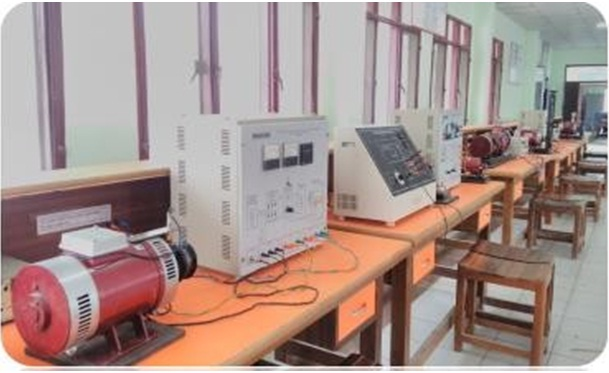• 8070500600, 9027916050, 9027916051
• Apply Now# Electrical & Electronics Laboratories### Electrical & Electronics Engineering Department

Electrical Engineering is a subject that needs theoretical as well as practical expertise to be a good electrical engineer. Hence department of Electrical and Electronics Engineering is equipped with world class laboratories with all automated instruments.

### Basic Electrical and Electronics Engineering Laboratory

The Basic Electrical and Electronics Engineering laboratory is utilized for performing experiments related to fundamentals of the subject common to all first-year students .

Electrical and Electronics Engineering, a core discipline, is devoted to study the principles of electricity to develop machines, devices and systems. The study of electrical and electronic is pivotal to both, electrical and electronics engineering students.This lab covers all the basic devices that serve as the most basic building block of almost all the electronic and electrical devices. This lab lays a firm foundation of what is taught throughout the engineering curriculum.

Outcomes: The students are expected to

• Get an exposure to common electrical components and their ratings. Make electrical connections by wires of appropriate ratings.
• Understand the usage of common electrical measuring instruments.
• Understand the basic characteristics of transformers and electrical machines. Get an exposure to the working of power electronic converters.### Networks Analysis and Synthesis Laboratory

The network analysis lab is a very basic skill set needed by all who want to do major in Electronics,instrumentation or electrical engineering. This lab aims at equipping students with the analysis and techniques, which will be used throughout the engineering curriculum. This lab develops a general aptitude expected out of engineering students, by virtue of which they can understand and analyze basic electrical and electronic circuits.

Outcomes: The students will be able to understand

• Basic electrical circuits with nodal and mesh analysis.
• Electrical network theorems.
• Steady state and transient analysis.
• Frequency response of current in RLC circuit with sinusoidal ac input.
• Z and H parameters (dc only) for a network and computation of Y and ABCD parameters.
• Driving point and transfer functions of a two-port ladder network and verify with theoretical values.### Electrical Measurements & Instrumentation Laboratory

This laboratory focuses on training the second year students about measuring techniques. This lab is used for measuring different parameters like temperature, pressure, strain, and speed along with measurement of resistance, inductance, capacitance and frequency. The lab consists of different measuring instruments like thermocouple module, pressure transducer module, strain gauge module, magnetic sensor and photoelectric sensor and various bridge circuits. This lab is adequately equipped with function generators ,CROs,DSOs,voltmeters,ammeters,multi-meters etc.

Outcomes: The students are expected

• To understand the importance of calibration of measuring instruments
• To understand and learn the construction and working of different measuring instruments
• To understand and learn the construction and working of different AC and DC bridges, along with their applications.
• To measure electrical engineering parameters like voltage, current, power & phase difference in industry as well as in power generation, transmission and distribution sectors.
• To understand and acquire the capability to analyze and solve the variety of problems in the field of electrical measurements.### Electrical Machine Laboratory

Electromechanical Energy Conversion (EMEC) Laboratory is one of the oldest and important laboratory of Electrical Engineering Department. The lab is equipped with conventional and modern electrical & electronic measuring equipments and AC &DC electrical machines. Various test setups are as follows:

• Synchronous Motors
• Alternators
• Transformers
• DC Motors
• Generators

Outcomes: The students are expected

• To understand various tests on transformers.
• To understand the electrical parameters, efficiency, and regulation of transformers.
• To study polarity and ratio test of single phase and 3‐phase transform
• To understand the operation and characteristics of DC Machines.
• To understand Hopkinson’s test and determine losses and efficiency of DC machine.

### Power System-I Laboratory

Power system lab is equipped with apparatus and devices to make all important tests of power system including generation unit with control panel and protection equipment's passing through the transmission lines to the load units   with power factor correction panel. Various experimental setups are as follows :

• Dielectric strength of transformer oil
• Thermal relays characteristic
• IDMT Relay
• Fault detection inline
• ABCD parameters offline

Outcomes: The students are expected to

• To develop MATLAB Program to Simulate Ferranti Effect
• To understand MATLAB Program to Model Transmission Lines
• To observe the voltage distribution across an Insulator String
• To understand Sag and factors effecting on Sag of Transmission Line
• To understand Three phase short circuit analysis in a Synchronous Machine using MATLAB/ SIMULINK.

### Control system Laboratory

Control system is a very important subject for electrical engineers. They get the knowledge on applications of machines & electronic devices with control systems. Various experimental setups are used to find the transfer function of the system for controlling. Few experimental setups are as follows :

• PID control of oventemp
• Synchro transmitter & receiver for positionerror
• Position error detector
• DC speedcontrol
• LVDT, RTD

Outcomes: The students are expected to

• Analyze the use of various error detectors in control systems and distinguish between each one of them.
• Comprehend and evaluate the performance of various position control systems.
• Build, simulate and analyze different control systems for their time responses and frequency responses using MATLAB.
• Compare different speed control mechanism used to control the speed of various servomotors.

### Power Electronics Laboratory

Knowledge of power electronics is essential to make the students understand various power electronics devices such as SCR, TRIAC, DIAC, IGBT, and GTO etc. , application of aforesaid Power Electronics devices in Choppers, Inverters and Converters etc. and control of Electrical Motors through DC-DC converters, AC Converters etc.

Various experimental setups are as follows.

• SCRcharacteristic
• Triggering of SCR
• Phase Control circuit of SCR
• Half & full control bridgerectifier

Outcomes: The students are expected to

• Deduce the characteristics of Power Electronic switches with various parameters.
• Study and analyze power electronic converters.
• Simulate and analyze various power electronic circuits.

# Electric Drive Laboratory

Main focus of the laboratory is on the measurement and control of electric drives. This lab enables students to understand the implementation of power electronics in controlling of electrical machine. Various schemes of control of drives of single & three phase induction & DC motor drive various experimental setups are as follows:

• Speed control of DC motor using dual converter
• Speed control using IGBT
• Slip power recovery scheme